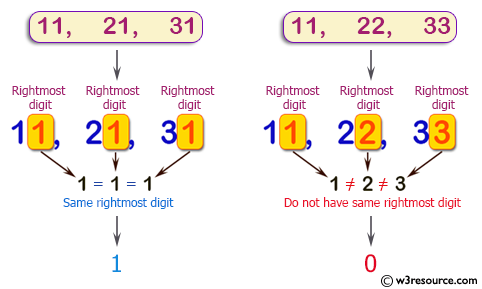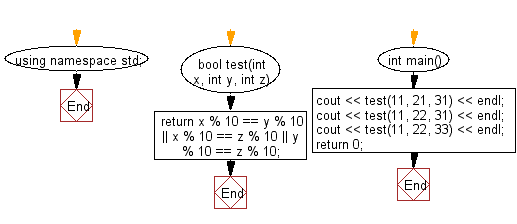﻿ C++ : Compare the rightmost digits of non-negative integers# C++ Exercises: Check if two or more non-negative given integers have the same rightmost digit

## C++ Basic Algorithm: Exercise-47 with Solution

Write a C++ program to check if two or more non-negative given integers have the same rightmost digit.

Sample Solution:

C++ Code :

``````#include <iostream>

using namespace std;

bool test(int x, int y, int z)
{
return x % 10 == y % 10 || x % 10 == z % 10 || y % 10 == z % 10;
}

int main()
{
cout << test(11, 21, 31) << endl;
cout << test(11, 22, 31) << endl;
cout << test(11, 22, 33) << endl;
return 0;
}
``````

Sample Output:

```1
1
0
```

Pictorial Presentation:Flowchart:C++ Code Editor: